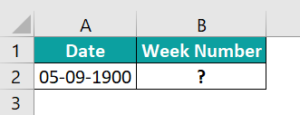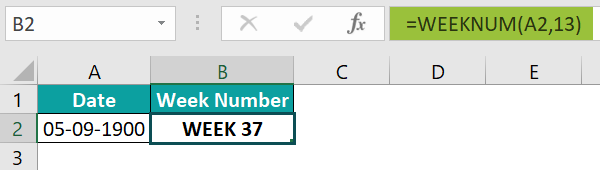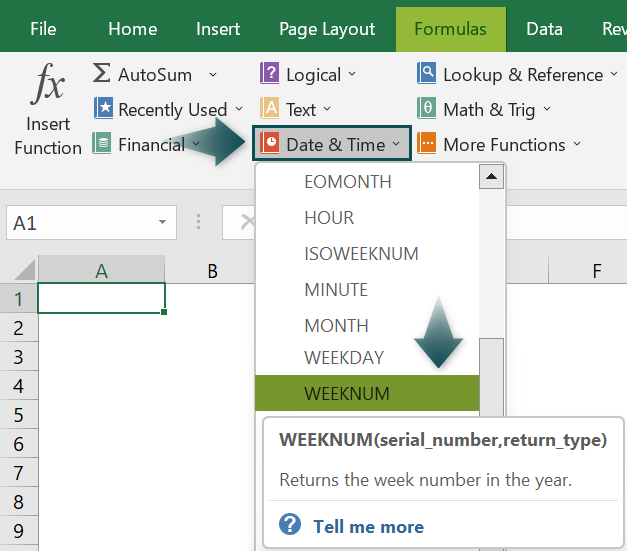# Week Number in ExcelArticle byExcelMojo Team## What Is Week Number In Excel?

The Week Number or WEEKNUM function is a Date function in Excel that evaluates the week number for the entered date. This function checks the date as an argument which can be a date or a serial number, and also represents the day the week begins.

The WEEKNUM excel function is categorized under the functional library’s Date and Time function group.

For example, we will calculate the week number from a given date using the WEEKNUM functions in Excel.

The WEEKNUM formula is entered in cell B2; the complete formula is =WEEKNUM(A2,1), and the result is returned as ‘53.’

###### Key Takeaways
• The Week Number in Excel calculates the weeks from the given dates.
• The WEEKNUM function is a Date function in Excel that evaluates week number for the entered date.
• The syntax of the WEEKNUM Excel Function is WEEKNUM(serial_number,[return_type]), where,
• serial_number is the mandatory argument and denotes a date.
• return_type is the optional argument and indicates the start of the weekday.
• The WEEKNUM function used in Week Number in Excel.
• The WEEKNUM function is the family member of Excel’s DATE, DAY, MONTH, YEAR, and WEEKDAY functions.

### Formula

The Formula of the WEEKNUM Excel Function is

• serial_number: This is the mandatory argument and denotes a date. Excel treats date as a serial number.
• return_type: This is the optional argument and indicates the start of the weekday.

### How To Use The Week Number (WEEKNUM) Function In Excel?

#### #1 – Access From The Excel Ribbon

1. Choose the empty cell which will contain the result.
2. Go to the Formulas tab and click it.
3. Select the Date & Time option from the menu.
4. Select WEEKNUM from the drop-down menu.
1. A window called Function Arguments appears.
2. As the number of arguments, enter the value in the serial_number and return_type.
3. Select OK.

#### #2 – Enter The Worksheet Manually

1. Select an empty cell for the output.
2. Type =WEEKNUM( in the selected cell. Alternatively, type =W and double-click the WEEKNUM function from the list of suggestions shown by Excel.
3. Press Enter key.

### Example #1 – Finding Week Number In Excel

In the following example, we will calculate the week number from given dates using the WEEKNUM functions in Excel.

In the table,

• Column A contains the Date.
• Column B contains the Week Number.

The steps to calculate the week number by the WEEKNUM function in Excel are as follows:

• Step 1: First, select the column where we will enter the formula and then, calculate the result. The selected cell, in this case, is cell B2.
• Step 2: Next, we will enter the Excel WEEKNUM Formula in cell B2.
• Step 3: Then, enter the value of the serial number argument as A2, i.e., date value cell A2.
• Step 4: Now, enter the return type value as 1, i.e., 1 for Sunday.
• Step 5: So, the complete formula entered is =WEEKNUM(A2,1).
• Step 6: Next, press the Enter key. The result is obtained in cell B2 ass 22.
• Step 7: Now, press Enter and then, drag the cursor to cell B6, as shown in the following image.

### Example #2 – Add The Word WEEK To The Week Number In Excel

In the following example, we will calculate the week number and add the word WEEK to the Week Number from given dates using the WEEKNUM functions in Excel.

In the table,

• Column A contains the Date.
• Column B contains the Week Number.

The steps to calculate the week number by the WEEKNUM function in Excel are as follows:

• Step 1: Select the column where we will enter the formula and calculate the result. The selected cell, in this case, is cell B2.
• Step 2: Next, we will enter the Excel WEEKNUM Formula in cell B2.
• Step 3: Enter the value of the serial number argument as A2, i.e., date value cell A2.
• Step 4: Enter the return type value as 2, i.e., 2 for Monday.
• Step 5: The complete formula entered is =WEEKNUM(A2,2).
• Step 6: Press Enter key. The results in cell B2 is obtained as 36.
• Step 7: Press Enter and drag the cursor to cell B6, as shown in the following image.
• Step 8: Copy the calculated Week Number to column C.
• Step 9: Select the cells containing output, and then right-click.
• Step 11: The window called the Format Cells opens.
• Step 12: Select the Custom option from the category list of the Number menu.
• Step 13: Change the Type: of the format to the WEEK.
• Step 14: Click OK.
• Step 15: The Word WEEK is added to the Week Number in Excel, as shown in the below image.

### Example #3 – Calculate Number Of Weeks In Excel Between Two Dates

In the following example, we will calculate the number of weeks between the two dates using the Excel formula.

In the table,

• Column A contains the Date 1.
• Column B contains the Date 2.
• Column C contains the Week Number.

The steps to calculate the week number between the two dates are as follows:

• Step 1: Select the column where we will enter the formula and calculate the result. The selected cell, in this case, is cell C2.
• Step 2: Next, we will enter the Excel Formula in cell C2.
• Step 3: The complete formula entered is =(B2-A2)/7.
• Step 4: Press Enter key. The results in cell C2 is returned as 4.

### Example #4 – Calculate The Number Of Weeks in Fraction Using Excel

In the following example, we will calculate the number of weeks between the two dates in fractions using the Excel formula.

In the table,

• Column A contains the Date 1.
• Column B contains the Date 2.
• Column C contains the Week Number.

The steps to calculate the week number between the two dates are as follows:

• Step 1: Select the column where we will enter the formula and calculate the result. The selected cell, in this case, is cell C2.
• Step 2: Next, we will enter the Excel Formula in cell C2.
• Step 3: The complete formula entered is =(B2-A2)/7.
• Step 4: Press Enter. The results are obtained as shown in the image below.
• Step 5: Select the cells containing output, and then right-click.
• Step 6: Select the Format Cells… from the list that pops up.
• Step 7: The window called the Format Cells opens.
• Step 8: Select the Fraction option from the category list of the Number menu.
• Step 9: Change the Type: of the format to the As quarters (2/4).
• Step 10: Click OK.
• Step 11: The number of weeks between the two dates in fractions is calculated, as shown in the below image.

### Important Things To Note

• The week starts in Excel by SUNDAY.
• The return type should be entered correctly for a different week.
• The “#VALUE!” error occurs when the date is not in the correct format.

1. WEEKNUM function in Excel definition?

The WEEKNUM function in Excel calculates and returns the value representing the number of weeks from a given date. The WEEKNUM function works with dates entered as; a result of some other function, dates stored as text, or dates entered as text in the WEEKNUM function.

The formula of the WEEKNUM function in Excel is as follows;
=WEEKNUM(serial_number,[return_type])

2. How does the WEEKNUM function work in Excel?

The WEEKNUM function works in Excel using the following steps;
Select an empty cell to enter the Formula.
Type =WEEKNUM( in the selected cell. Alternatively, type =W and double-click the WEEKNUM function from the list of suggestions shown by Excel.
Press Enter key.

In the following example, we will calculate the week number from a given date using the WEEKNUM functions in Excel.The steps to calculate the weekday by the WEEKNUM function in Excel are as follows:

• Step 1: Select the column where we will enter the formula and calculate the result. The selected cell, in this case, is cell B2.

• Step 2: Next, we will enter the Excel WEEKNUM Formula in cell B2.

• Step 3: Enter the value of the serial number argument as A2, i.e., date value cell A2.

• Step 4: Enter the return type value as 13, i.e., 13 for Wednesday.

• Step 5: The complete formula entered is =WEEKNUM(A2,13).

• Step 6: Press Enter. The result is obtained as shown in the image below.3. Where is the WEEKNUM formula in Excel?

One can activate the WEEKNUM function in Excel using the following steps:
Choose the empty cell which will contain the result.
Go to the Formulas tab and click it.
Select the Date & Time group.
Select WEEKNUM from the drop-down menu.
A window called Function Arguments appears.
As the number of arguments, enter the value in the serial_number and return_type.
Select OK.